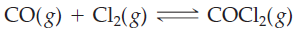×
Get Full Access to Introductory Chemistry - 5 Edition - Chapter 15 - Problem 63p
Get Full Access to Introductory Chemistry - 5 Edition - Chapter 15 - Problem 63p

×

# Solution: Consider this reaction at equilibrium. Predict the effect (shift right, shiftISBN: 9780321910295 34

## Solution for problem 63P Chapter 15

Introductory Chemistry | 5th Edition

• Textbook Solutions
• 2901 Step-by-step solutions solved by professors and subject experts
• Get 24/7 help from StudySoup virtual teaching assistantsIntroductory Chemistry | 5th Edition

4 5 1 342 Reviews
27
4
Problem 63P

Consider this reaction at equilibrium.Predict the effect (shift right, shift left, or no effect) of these changes.

(a) adding Cl2 to the reaction mixture

(b) adding COCl2 to the reaction mixture

(c) adding CO to the reaction mixture

Step-by-Step Solution:

Solution 63P :

Step 1:

Given reaction at equilibrium.

CO2(g) + Cl2(g) ⇋COCl2(g)

Le Chatelier's principle is also called as ‘The equilibrium law’. It states that ‘ The changes to an equilibrium system will result in a predictable shift that will counteract the change’. It is used to predict the changes in system that is caused due to temperature, pressure, volume or concentration.

According to Le Chatelier's principle :

• When a chemical system at equilibrium is disturbed, the system shifts in a direction that minimizes the disturbance
• Adding additional reactant to a system will shift the equilibrium to the right which is towards the side of the products
• Removing the reactants from the system will cause the equilibrium to shift to the left which is towards reactant.
• Similarly, adding additional product to a system will shift the equilibrium to the left, in order to produce more reactants.
• Reducing the concentration of product will shift the equilibrium to the right, towards the products.

Step 2 of 2

##### ISBN: 9780321910295

Unlock Textbook Solution